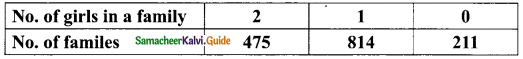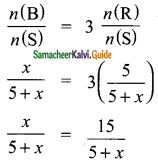Students can download Maths Chapter 9 Probability Additional Questions and Answers, Notes, Samacheer Kalvi 9th Maths Guide Pdf helps you to revise the complete Tamilnadu State Board New Syllabus, helps students complete homework assignments and to score high marks in board exams.

## Tamilnadu Samacheer Kalvi 9th Maths Solutions Chapter 9 Probability Additional Questions

Question 1.
Which of the following cannot be taken as probability of an event?
(a) 0
(b) 0.35
(c) $$\frac{7}{20}$$
(d) –$$\frac{7}{20}$$
Solution:
(d) –$$\frac{7}{20}$$

Question 2.
A letter is chosen at random from the word “MATHEMATICS” the probability of getting a vowel is ……..
(a) $$\frac{2}{11}$$
(b) $$\frac{3}{11}$$
(c) $$\frac{4}{11}$$
(d) $$\frac{5}{11}$$
Solution:
(c) $$\frac{4}{11}$$Question 3.
If P(A) =$$\frac{1}{3}$$ then P(A)’ is ………
(a) $$\frac{1}{3}$$
(b) $$\frac{2}{3}$$
(c) $$\frac{3}{2}$$
(d) 1
Solution:
(b) $$\frac{2}{3}$$

Question 4.
An integer is chosen from the first twenty natural number, the probability that it is a prime number is ……..
(a) $$\frac{1}{5}$$
(b) $$\frac{2}{5}$$
(c) $$\frac{3}{5}$$
(d) $$\frac{4}{5}$$
Solution:
(b) $$\frac{2}{5}$$

Question 5.
From a well shuffled pack of 52 cards one card is drawn at random. The probability of getting not a king is ………
(a) $$\frac{12}{13}$$
(b) $$\frac{1}{13}$$
(c) $$\frac{4}{13}$$
(d) $$\frac{2}{13}$$
Solution:
(a) $$\frac{12}{13}$$Question 6.
1500 families with 2 children were selected randomly and the following data were recorded.Compute the probability of a family chosen at random having one girl.
Solution:
Total number of families = 1500
∴ n(S) = 1500
Let E be the event of getting one girl
n(E) = 814
P(E) = $$\frac{n(E)}{n(S)}$$
= $$\frac{814}{1500}$$
= $$\frac{407}{750}$$Question 7.
The record of weather station shows that out of the part 250 consecutive days its whether forecast were correct 175 time. What is the probability that it was not correct on a given data?
Solution:
n(S) = 250
Let E be the event of getting whether forecast were correct
n(E) = 175
P(E) = $$\frac{n(E)}{n(S)}$$
= $$\frac{175}{250}$$
= $$\frac{7}{10}$$
Forecast was not correct on a given day = 1 – P(E)
= 1 – $$\frac{7}{10}$$
= $$\frac{10-7}{10}$$
= $$\frac{3}{10}$$Question 8.
If A coin is tossed 200 times and is found that a tail comes up for 120 times. Find the probability of getting a tail.
Solution:
Number of trials = 200
n(S) = 200
Let E be the event of getting a tail
n(E) = 120
p(E) = $$\frac{n(E)}{n(S)}$$
= $$\frac{120}{200}$$
= $$\frac{12}{20}$$
= $$\frac{3}{5}$$

Question 9.
A bag contains 5 red balls and some blue balls. If the probability of drawing a blue ball from the bag is thrice that of drawing a red ball, then find the number of blue balls in the bag.
Solution:
Let the number of blue balls be “x”
Total number of balls = 5 + x
∴ n(S) = 5 + x
Let B be the event of drawing a blue ball and R be the event of drawing a red ball
Given P(B) = 3P(R)∴ x = 15
Number of blue balls = 15Question 10.
Find the probability that a non leap year selected at random will have 53 fridays.
Solution:
No. of days in a non leap year = 365 days
This year contain 52 weeks and one day
Sample space = {Sun, Mon, Tue, Wed, Thu, Fri, Sat}
n(S) = 7
Let A be the event of getting a friday
n(A) = 1
P(A) = $$\frac{n(A)}{n(S)}$$
= $$\frac{1}{7}$$